TitleCollege Algebra
Answer/Discussion to Practice Problems
Tutorial 14: Linear Equations in One Variable

WTAMU > Virtual Math Lab > College Algebra > Tutorial 14: Linear Equations in One VariableAnswer/Discussion to 1a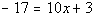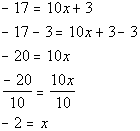*Inverse of add. 3 is sub 3 from both sides   *Inverse of mult. by 10 is div. both sides by 10

 If you put -2 back in for x in the original problem you will see that -2 is the solution we are looking for.Answer/Discussion to 1b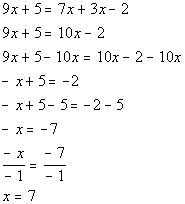*Get all x terms on one side *Inverse of add. 5 is sub. 5   *Inverse of mult. by -1 is div. by -1

 If you put 7 back in for x in the original problem you will see that 7 is the solution we are looking for.Answer/Discussion to 1c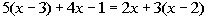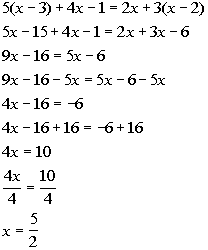*Remove ( ) by using dist. prop. *Combine like terms *Get all x terms on one side *Inverse of sub. 16 is add. 16     *Inverse of mult. by 4 is div. by 4

 If you put 5/2 back in for x in the original problem you will see that 5/2 is the solution we are looking for.Answer/Discussion to 2a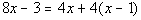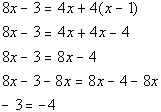*Remove ( ) by using dist. prop. *Combine like terms *Get all the x terms on one side

 Where did our variable, x, go???  It disappeared on us.  Also note how we ended up with a FALSE statement, -3 is not equal to -4.  This does not mean that x = -3 or x = -4.  Whenever your variable drops out AND you end up with a FALSE statement, then after all of your hard work, there is NO SOLUTION. So, the answer is no solution which means this is an inconsistent equation.Answer/Discussion to 2b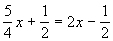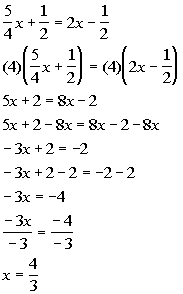*To get rid of the fractions,  mult. both sides by the LCD of 4       *Get all the x terms on one side   *Inverse of add. 2 is sub. 2     *Inverse of mult. by -3 is div. by -3

 If you put 4/3 back in for x in the original problem you will see that 4/3  is the solution we are looking for. This would be an example of a conditional equation, because we came up with one solution.Answer/Discussion to 2c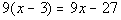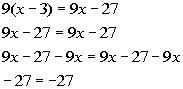*Remove ( ) by using dist. prop. *Get all the x terms on one side

 Where did our variable, x, go???  It disappeared on us.  Also note how we ended up with a TRUE statement, -27 does indeed equal -27.  This does not mean that x = -27.  Whenever your variable drops out AND you end up with a TRUE statement, then the solution is ALL REAL NUMBERS. This means that if you plug in any real number for x in this equation, the left side will equal the right side. So the answer is all real numbers, which means this equation is an identity.

WTAMU > Virtual Math Lab > College Algebra > Tutorial 14: Linear Equations in One Variable

Last revised on Dec. 16, 2009 by Kim Seward.
All contents copyright (C) 2002 - 2010, WTAMU and Kim Seward. All rights reserved.